1. /
2. CBSE
3. /
4. Class 12
5. /
6. Physics
7. /
8. NCERT Solutions class 12...

# NCERT Solutions class 12 physics Atoms### myCBSEguide App

Download the app to get CBSE Sample Papers 2023-24, NCERT Solutions (Revised), Most Important Questions, Previous Year Question Bank, Mock Tests, and Detailed Notes.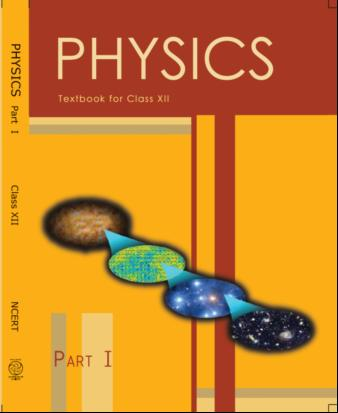## NCERT Class 12 Physics Chapter-wise Solutions

• 1 – Electric Charges and Fields
• 2 – Electrostatic Potential and Capacitance
• 3 – Current Electricity
• 4 – Moving Charges and Magnetism
• 5 – Magnetism and Matter
• 6 – Electromagnetic Induction
• 7 – Alternating Current
• 8 – Electromagnetic Waves
• 9 – Ray Optics and Optical Instruments
• 10 – Wave Optics
• 11 – Dual Nature of Radiation and Matter
• 12 – Atoms
• 13 – Nuclei
• 14 – Semiconductor Electronic: Material, Devices and Simple Circuits
• 15 – Communication Systems

## CHAPTER 12 ATOMS

• 12.1 Introduction
• 12.2 Alpha-particle Scattering and Rutherford’s Nuclear Model of Atom
• 12.3 Atomic Spectra
• 12.4 Bohr Model of the Hydrogen Atom
• 12.5 The Line Spectra of the Hydrogen Atom
• 12.6 DE Broglie’s Explanation of Bohr’s Second Postulate of Quantisation

## NCERT Solutions class 12 physics Atoms

1:  Choose the correct alternative from the clues given at the end of the each statement:

(a) The size of the atom in Thomson’s model is ………. the atomic size in Rutherford’s model. (much greater than/no different from/much less than.)

(b) In the ground state of ………. electrons are in stable equilibrium, while in ………. electrons always experience a net force.

(Thomson’s model/ Rutherford’s model.)

(c) A classical atom based on ………. is doomed to collapse.

(Thomson’s model/ Rutherford’s model.)

(d) An atom has a nearly continuous mass distribution in a ………. but has a highly non-uniform mass distribution in ……….

(Thomson’s model/ Rutherford’s model.)

(e) The positively charged part of the atom possesses most of the mass in ………. (Rutherford’s model/both the models.)

2: Suppose you are given a chance to repeat the alpha-particle scattering experiment using a thin sheet of solid hydrogen in place of the gold foil. (Hydrogen is a solid at temperatures below 14 K.) What results do you expect?

3:  What is the shortest wavelength present in the Paschen series of spectral lines?

4:  A difference of 2.3 eV separates two energy levels in an atom. What is the frequency of radiation emitted when the atom makes a transition from the upper level to the lower level?

5: The ground state energy of hydrogen atom is -13.6 eV. What are the kinetic and potential energies of the electron in this state?

6:  A hydrogen atom initially in the ground level absorbs a photon, which excites it to the n = 4 level. Determine the wavelength and frequency of the photon.

7: (a) Using the Bohr’s model calculate the speed of the electron in a hydrogen atom in the n = 1, 2, and 3 levels. (b) Calculate the orbital period in each of these levels.

8: The radius of the innermost electron orbit of a hydrogen atom is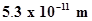. What are the radii of the n = 2 and n =3 orbits?

9: A 12.5 eV electron beam is used to bombard gaseous hydrogen at room temperature. What series of wavelengths will be emitted?

10: In accordance with the Bohr’s model, find the quantum number that characterises the earth’s revolution around the sun in an orbit of radius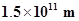with orbital speed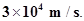(Mass of earth =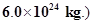11:  Answer the following questions, which help you understand the difference between Thomson’s model and Rutherford’s model better.

(a) Is the average angle of deflection of α­-particles by a thin gold foil predicted by Thomson’s model much less, about the same, or much greater than that predicted by Rutherford’s model?

(b) Is the probability of backward scattering (i.e., scattering of α-particles at angles greater than 90°) predicted by Thomson’s model much less, about the same, or much greater than that predicted by Rutherford’s model?

(c) Keeping other factors fixed, it is found experimentally that for small thickness t, the number of α-particles scattered at moderate angles is proportional to t. What clue does this linear dependence on t provide?

(d) In which model is it completely wrong to ignore multiple scattering for the calculation of average angle of scattering of α-particles by a thin foil?

12:  The gravitational attraction between electron and proton in a hydrogen atom is weaker than the coulomb attraction by a factor of about 10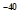. An alternative way of looking at this fact is to estimate the radius of the first Bohr orbit of a hydrogen atom if the electron and proton were bound by gravitational attraction. You will find the answer interesting.

13:  Obtain an expression for the frequency of radiation emitted when a hydrogen atom de-excites from level n to level (n-1). For large n, show that this frequency equals the classical frequency of revolution of the electron in the orbit.

14: Classically, an electron can be in any orbit around the nucleus of an atom. Then what determines the typical atomic size? Why is an atom not, say, thousand times bigger than its typical size? The question had greatly puzzled Bohr before he arrived at his famous model of the atom that you have learnt in the text. To simulate what he might well have done before his discovery, let us play as follows with the basic constants of nature and see if we can get a quantity with the dimensions of length that is roughly equal to the known size of an atom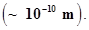(a) Construct a quantity with the dimensions of length from the fundamental constants e, me, and c. Determine its numerical value.

(b) You will find that the length obtained in (a) is many orders of magnitude smaller than the atomic dimensions. Further, it involves c. But energies of atoms are mostly in non-relativistic domain where c is not expected to play any role. This is what may have suggested Bohr to discard c and look for ‘something else’ to get the right atomic size. Now, the Planck’s constant h had already made its appearance elsewhere. Bohr’s great insight lay in recognising that h, me, and e will yield the right atomic size. Construct a quantity with the dimension of length from h, me, and e and confirm that its numerical value has indeed the correct order of magnitude.

15:  The total energy of an electron in the first excited state of the hydrogen atom is about -3.4 eV.

(a) What is the kinetic energy of the electron in this state?

(b) What is the potential energy of the electron in this state?

(c) Which of the answers above would change if the choice of the zero of potential energy is changed?

16:  If Bohr’s quantisation postulate (angular momentum = nh/2π) is a basic law of nature, it should be equally valid for the case of planetary motion also. Why then do we never speak of quantisation of orbits of planets around the sun?

17:  Obtain the first Bohr’s radius and the ground state energy of a muonic hydrogen atom [i.e., an atom in which a negatively charged muon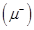of mass about 207me orbits around a proton].

## NCERT Solutions for Class 12 Physics

NCERT Solutions Class 12 Physics PDF (Download) Free from myCBSEguide app and myCBSEguide website. Ncert solution class 12 physics includes text book solutions from both part 1 and part 2. NCERT Solutions for CBSE Class 12 Physics have total 20 chapters. 12 Physics NCERT Solutions in PDF for free Download on our website. Ncert physics class 12 solutions PDF and physics ncert class 12 PDF solutions with latest modifications and as per the latest CBSE syllabus are only available in myCBSEguide

To download NCERT Solutions for class 12 Physics, Chemistry, Biology, History, Political Science, Economics, Geography, Computer Science, Home Science, Accountancy, Business Studies and Home Science; do check myCBSEguide app or website. myCBSEguide provides sample papers with solution, test papers for chapter-wise practice, NCERT solutions, NCERT Exemplar solutions, quick revision notes for ready reference, CBSE guess papers and CBSE important question papers. Sample Paper all are made available through the best app for CBSE students and myCBSEguide website.### Test Generator

Create question paper PDF and online tests with your own name & logo in minutes.### myCBSEguide

Question Bank, Mock Tests, Exam Papers, NCERT Solutions, Sample Papers, Notes